# Feature Space Regularization for Person Re-Identification with One Sample

## Few-shot Person Re-ID

Posted by JoselynZhao on July 5, 2020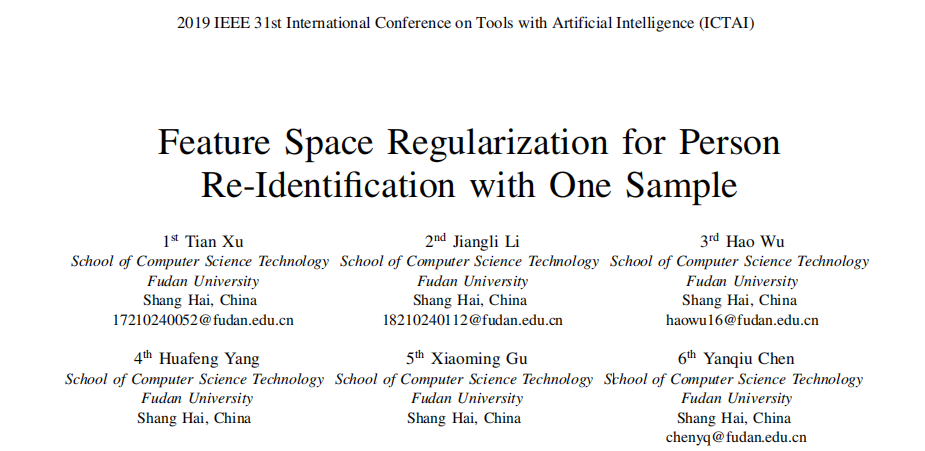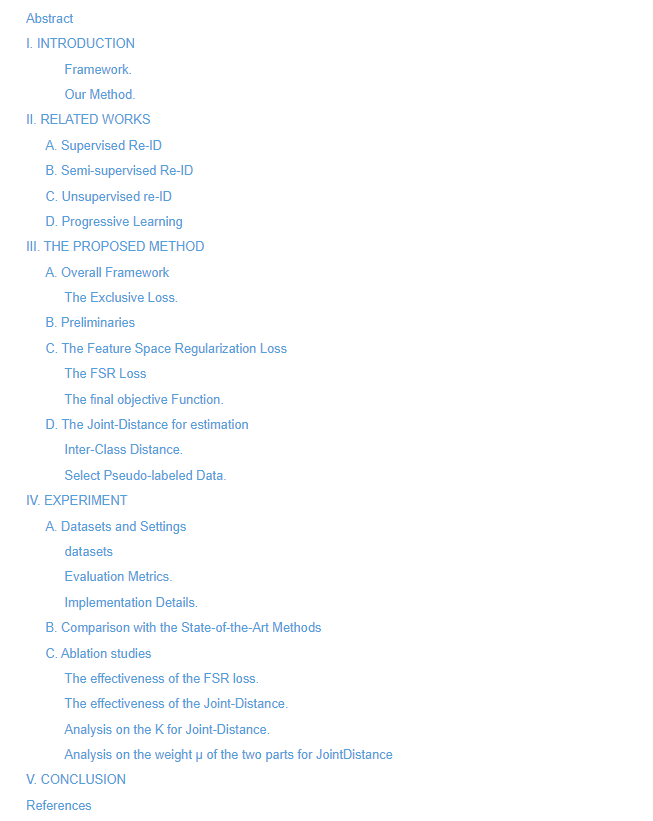# Abstract

Targeting to solve the issues above, we propose two simple and effective solutions.

• (a) We design the Feature Space Regularization (FSR) Loss to adjust the distribution of samples in feature space.
• .(b)Wepropose combiningtheNearestNeighbordistancewithinter-classdistance to estimate pseudo-label for unlabeled data, which we called Joint-Distance.

Results：the Rank-1 accuracy of our method outperforms the state of the art method by a large margin of 12.1 points (absolute, i.e., 67.9% vs. 55.8%) on Market-1501, and 10.1 points (absolute, i.e., 58.9% vs. 48.8%) on DukeMTMCreID, respectively.

Code： FSR and JointDistance for ReID with one sample. （it hasn’t been made public yet

Index Terms : Person Re-Identiﬁcation, Few Shot Learning,One Shot Learning, Features Space Regularization,Joint Distance

# I. INTRODUCTION

Most of the existing methods adopt the supervised approach, which rely on a large amount of labeled data

The setup of this works:

• this work is devoted to the one sample learning setting in which only one labeled sample is needed of each identity.
• This paper adopts the same progressive learning strategy as in .

The key challenge for the one-shot image-based person ReID is the label estimation for the abundant unlabeled samples , .

**There are two main strategies to generating new training sets. **

• One type of approach is to use a static strategy to determine the quantity of selected pseudo-labeled data for further training.
• The other type of methods , , ,  adopt a progressive strategy to exploit the unlabeled data for training. The core idea of these methods comes from Curriculum Learning , which obtains knowledge from easy samples to hard samples in the training phase.

The problems of existing methods: training with a small number of samples will cause the model to be biased towards certain identities, which can be observed in Fig.1. This extremely unbalanced distribution of samples will lead to the selected pseudo-labeled are unbalanced for subsequent training.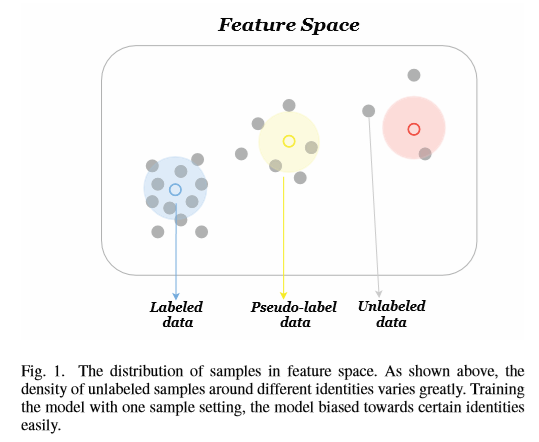### Framework.

two steps are involved:

1. using all the labeled and unlabeled data to train an initial model;
2. the initial model is used to estimate pseudo-label for all the unlabeled data and select some reliable samples as a new training set for next iteration.

The number of selected samples is controlled by the enlarged factor p.

### Our Method.

we propose the Feature Space Regularization loss to balance the distribution of samples in feature space and We use FSR to represent the Feature Space Regularization in the following content.

The FSR loss make the difference in distance between all labeled samples and unlabeled samples as small as possible, which can alleviate the samples imbalance during training to a certain extent.

We assume the K nearest neighbors of a unlabeled sample in unlabeled data set as the same identity, which is denoted as class U. Similarly, we can obtain the class L of a labeled sample. We combine the nearest class with nearest neighbor to estimate pseudo label for all unlabeled data.

Our contributions:

• We propose the Feature Space Regularization loss to balance the distribution of samples in feature space, which can help the model to learn a more robust representation.
• We design a new distance metric to estimate pseudo label for unlabeled data, which can get a higher prediction accuracy.
• Our method has achieved surprisingly superior performance on the one-shot learning in Re-ID, outperforming the state of the art method by 12.1 points on Market-1501 and 8.9 points on DukeMTMC-reID, the two large-scale datasets.

# II. RELATED WORKS

## A. Supervised Re-ID

The typical architecture is to use the classiﬁcation CNN to learn a robust representation for computing similarity score.

## B. Semi-supervised Re-ID

Semi-supervised learning usually combine labeled and unlabeled data to learn a robust model. Wu et al. ,  adopt the progressive learning, which gradually exploit the unlabeled data. Our work is based on  and achieve signiﬁcant progress.

## C. Unsupervised re-ID

Due to the unsupervised methods do not rely on labeled samples, the performance of these methods are poor relatively. In this work, we will pay our attention on one-shot learning.

## D. Progressive Learning

Most existing methods on one-shot setting adopt a progressive strategy, which obtain knowledge from easy to hard samples. The idea comes from the Curriculum Learning  (CL) proposed by Bengio et al.. Kumat et al. propose SelfPaced Learning (SPL)  which takes curriculum learning as a regularization term to update the model automatically.

# III. THE PROPOSED METHOD

Our method is based on the framework in .

## A. Overall Framework

the framework mainly consists of two steps:

1. train the model on labeled, pseudo-labeled, and unlabeled data by three loss functions
• Cross-Entropy Loss (CE Loss),
• Exclusive Loss (Ex Loss),
• Feature Space Regularization Loss (FSR Loss);
2. select a few reliable pseudo-labeled candidates from unlabeled data according to a certain strategy as a new subset for next training iteration.

The Exclusive Loss in  use unlabeled data as an auxiliary to improve training effect of the model.

### The Exclusive Loss.

The Ex loss learn a distinguishable feature by maximizing the distance of all unlabeled samples in feature space.

We denote the unlabeled samples set at tth iteration as $U^t, (x_i,x_j) ∈U^t$ and $i \ne j$. In addition, the CNN Extractor can be marked as $φ$, which well embed the images into the feature space.

The Ex loss can be described as follows: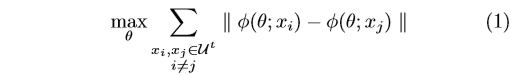The Ex loss can be approximately optimized by a softmax-like loss: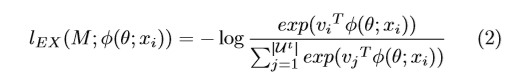where $v_i = φ(θ;x_i)$ be the L2-normalized feature embedding for the data $x_i$ and $M$ is the feature matrix of all the unlabeled data. More details can be obtained in .

## B. Preliminaries

Let $L =\left\{(x_1,y_1),...,(x_{n_l},y_{n_l})\right\}$ be the labeled set, and $U = \left\{(x_{n_l+1},...,(x_{n_l+n_u})\right\}$ be the unlabeled set, where $x_i$ and $y_i$ denotes the i-th image and its identity label, respectively. In addition, we have $\|L\|= n_l$ and $\|U\|= n_u$, where nl and nu are the number of samples. The CNN model $φ$ is used to embed images into the feature space.

For the evaluation stage： the query result is the ranking list of all gallery data according to the Euclidean Distance, i.e., $\| φ(θ;x_q) − φ(θ;x_g) \|$, where $x_q$ and $x_g$ denote the query data and the gallery data, respectively.

In estimation phase: we predict the pseudo label $\hat{y_i}$ for each unlabeled sample $x_i\in U$ and select a few reliable samples for the next iteration as in Fig.2.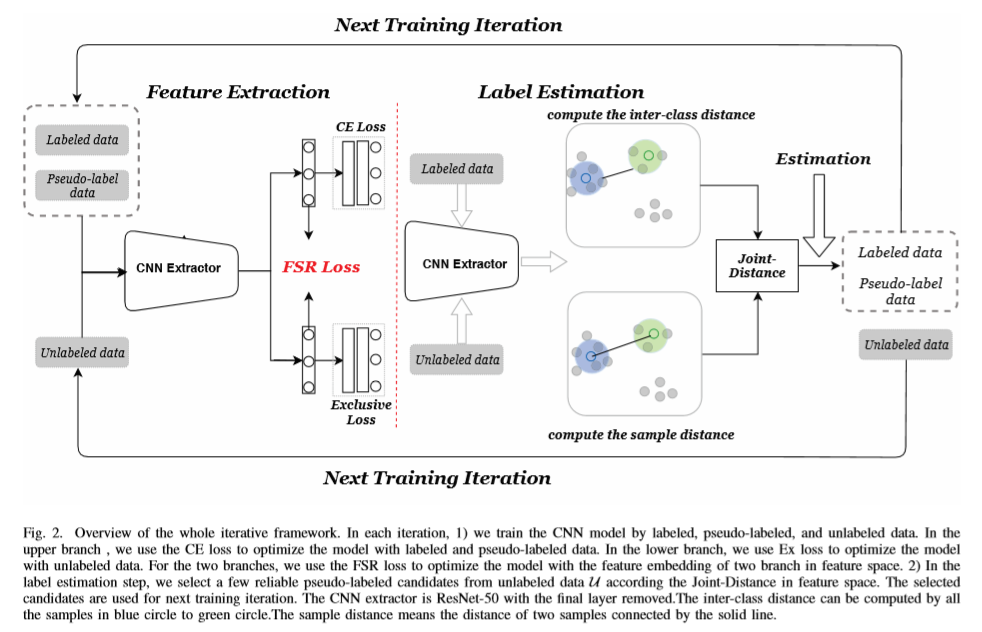We denote $S^t$ and $U^t$ as the pseudo-labeled dataset and unlabeled dataset at t-th step, respectively.

## C. The Feature Space Regularization Loss

Between the upper and lower branches in Fig.2, we utilize the FSR Loss to adjust distribution of the three types of data in feature space.

### The FSR Loss

In each forward propagation phase, a batch of labeled and pseudo-labeled data passing through the CNN model $φ$ will generate a batch of feature vectors, which can be marked as $V_l$. Similarly, a batch of feature vectors $V_u$ can also be obtained during the forward propagation with a batch of unlabeled samples.

For each $v_i \in V_l$, we will compute the distance as follows: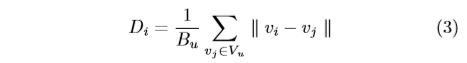where $B_u$ is the batchsize of unlabeled data and the $D_i$ means the average distance from vector $v_i$ to the whole $V_u$. Moreover, all the feature vectors are L2 normalized.

For $∀v_i ∈ V_l$, we can get the distance matrix D through Eq.(3), where $D_i ∈ D$. Based on D, we deﬁne the FSR loss as follows:where $\|·\|$means the Euclidean distance and $B_l$ is the batchsize of $V_l$. We calculate the sum of difference between any two samples according to the matrix D. By minimizing the $l_{FSR}$, we make the difference in distance between labeled samples and unlabeled samples smaller in feature space.

This balanced distribution will be proved to effective by the experiments.

### The ﬁnal objective Function.

For the labeled dataset $L$ and selected pseudo-labeled dataset $S_t$ where we have the identity (pseudo-)labels, we train the re-ID model as recent work , , . we have the following objective function:where $f(w;·)$ is an identity classiﬁer, parameterized by w, to classify the embedded feature $φ(θ;xi)$ into a k-dimension class estimation, in which k is the number of identities. $l_{CE}$ denotes the Cross-Entropy loss and $n_l,n_s$ denote the number of labeled and pseudo-labeled data at t-th step, respectively.

According to Eq.(2),(4),(5), we can get the ﬁnal objective function for the model training as t-th iteration as following: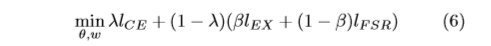where $λ,β$ are hyper-parameter to adjust the contribution of the three losses.

## D. The Joint-Distance for estimation

As is shown in Fig.3. The labels of $l_1$ and $l_2$ will be assigned to $u_1$ and $u_2$, respectively. Obviously, $u_1$ and $u_2$ are more likely belong to the same identity. Moreover, only distance between samples is used will be easily affected by outliers.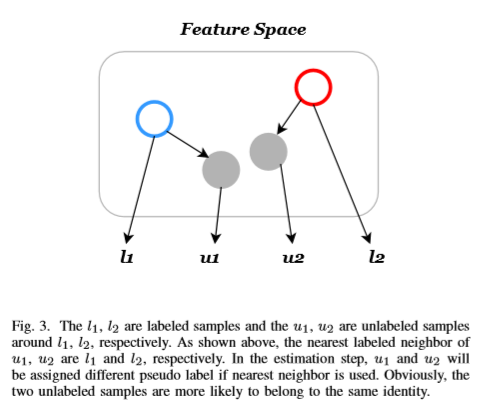### Inter-Class Distance.

We consider to utilize the unlabeled samples around the candidate as an auxiliary when measuring distance between samples.

We denote the K-Nearest Neighbors (KNN) of a certain labeled sample as $C_l$ and the K-Nearest Neighbors of a certain unlabeled candidate as $C_u$ in feature space. Intuitively, the samples of $C_l$ have a great possibility belong to one identity and $C_u$ is the same situation. We use $C_l$ to present the identity to which the labeled sample belongs and $C_u$ to present the identity to which the unlabeled candidate belongs, respectively. We deﬁne the Inter-Class Distance between a labeled sample and an unlabeled candidate according to the class $C_l$ and $C_u$ as follows:where $v_i ∈ C_l$ and $v_j ∈ C_u$ are embedded feature vector in feature space. Similarly, we can get the distance between each pair of labeled and unlabeled data: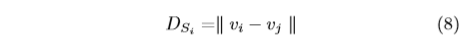According to Eq.(7),(8), we can get the two distance matrices, i.e., $D_c$ and $D_s$. $D_c$ and $D_s$ have the same size. For the two matrices, we utilize the min-max normalization to adjust the value of the matrices in range [0,1]. Finally, we can get the Joint-Distance matrix as follows: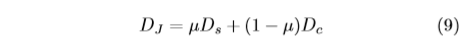where $μ$ controls the contribution of the two distance. Based onthedistancematrix $D_J$,we assign the label for all unlabeled data by its nearest labeled neighbor in $D_J$.

Compared to assign the pseudo-label for unlabeled data by the distance between samples, the Joint-Distance has taken the distance between classes into account, which is less affected by isolated sample.

### Select Pseudo-labeled Data.

For each unlabeled sample xi, we can get the distance $d_i ∈ D_J$ between $x_i ∈U^t$ and its nearest labeled neighbor in $D_J$. For all the unlabeled data, we select a certain number of samples based on distance di from small to large, which can be seen as a few reliable samples. The number of selected samples is controlled by enlarged factor p in .

# IV. EXPERIMENT

## A. Datasets and Settings

### datasets

We evaluate the proposed method on Market-1501 , and DukeM-TMC-reID , the two large-scale datasets with multiple cameras.

### Evaluation Metrics.

We report the Rank-1, Rank-5, Rank-10, Rank-20 scores to represent the CMC curve.

### Implementation Details.

• To optimize the model using FSR loss, we append an additional 1 × 1 conv layer with batch normalization.
• For the CE loss, we append an additional fullyconnected layer with batch normalization and a classiﬁcation layer on the upper branch in Fig.2.
• To optimize the model on unlabeled data by Ex loss, we append a fully-connected layer with batch normalization and a L2-normalization.
• We set the $λ,β$ to be 0.8 and $μ$ to be 0.5 for all the experiments.
• set λ =1, which means the unlabeled data is not used. For the label estimation stage, we set the K = 3 in all experiments.

## B. Comparison with the State-of-the-Art Methods

The re-ID performance of our method on the two large-scale datasets are summarized in Table 1 and Fig.4. Speciﬁcally, we achieve 12.1 and 10.1 points of Rank-1 accuracy improvement over the state of the art on Market-1501 and DukeMTMCreID,respectively. Our method is proved to be effective in different enlarged factor.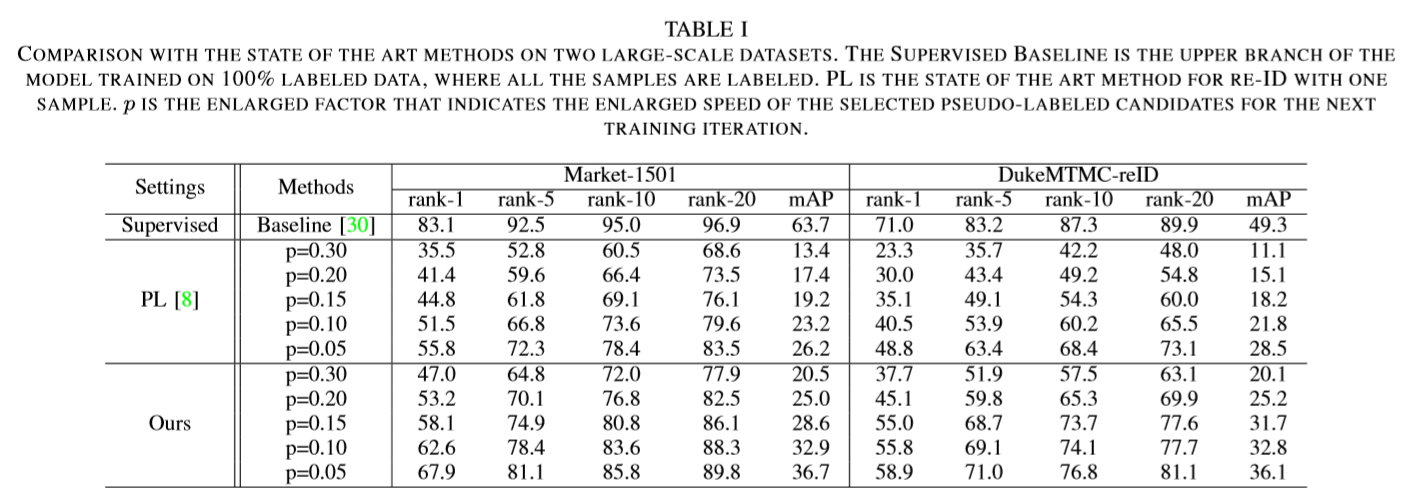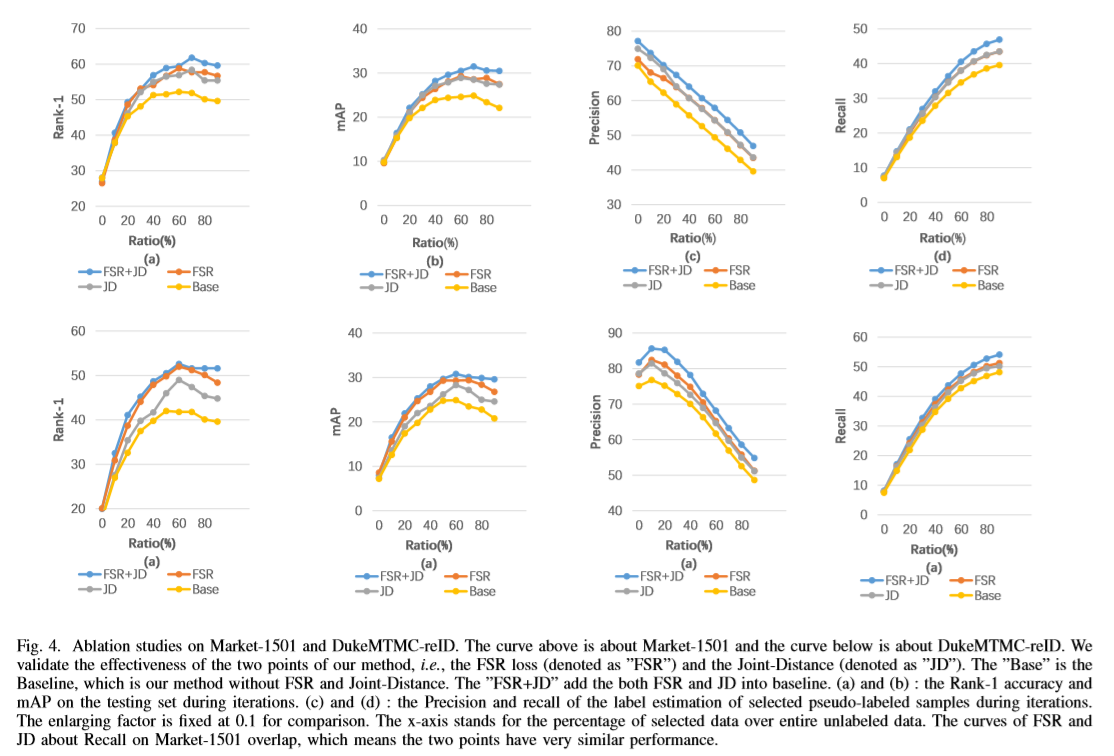## C. Ablation studies

### The effectiveness of the FSR loss.

To verify the effectiveness of the FSR loss, we conduct our method with only FSR loss, denoted as ”B + FSR” in Table 2 and Fig.4. The ”B” is the our method without both FSR and Joint-Distance, which has the same framework as .

As is shown in Table 2, the FSR has a better performance in any factor p and higher prediction accuracy, which means that the feature is more suitable to represent samples by feature space regularization .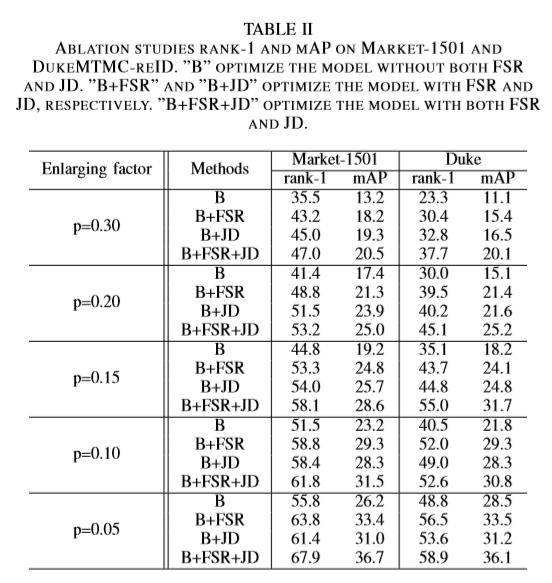### The effectiveness of the Joint-Distance.

We compare our method of Joint-Distance to the baseline in Table 2 and Fig.4. As shown in Fig.4, using the Joint-Distance has a higher prediction accuracy and recall in any iteration, which indicates that the Joint-Distance is more suitable for estimating pseudolabel than the nearest neighbor distance. Owing to the higher prediction accuracy of Joint-Distance, the model can has a better performance in rank-1 accuracy and mAP.

### Analysis on the K for Joint-Distance.

The value of K for K-NN is a key parameter in the Joint-Distance to estimate the pseudo-label. It controls the size of the inter-class for each sample. Smaller k indicates that we use fewer samples to present a class, which will belong to the same identity more possible. The results of different K on the two dataset can be found in Table 3.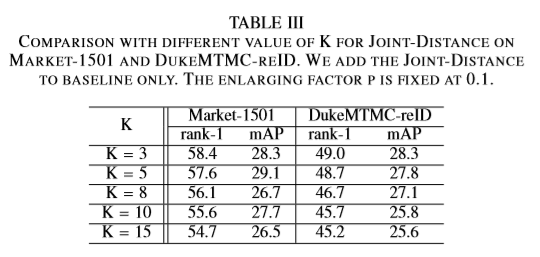As the K increasing, the rank-1 accuracy and mAP is gradually decreasing. The main reason is that a larger K value will result in more inaccurate samples in a class

### Analysis on the weight μ of the two parts for JointDistance

The weight μ of Joint-distance is a key parameter to estimate the pseudo-label. It controls the importance of the two parts of inter-class distance. The results of different μ on the two dataset can be found in Table 4.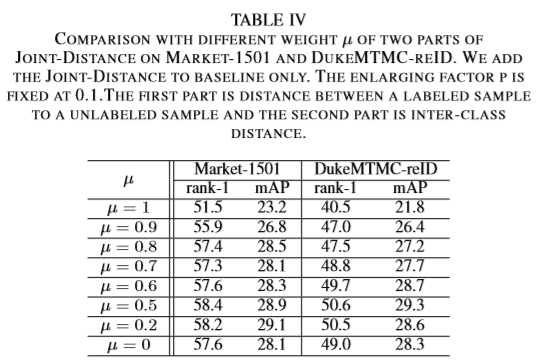As the μ decreasing, the rank-1 accuracy and mAP is gradually increasing and then decreasing. The main reason is that one part is too large or too small is not good for Joint-Distance to estimate the pseudolabel.Through the experiments,we can obtain that μ =0 .5 is a proper weight of the two parts of Joint-Distance and we use the 0.5 as the ﬁnal weight.

# V. CONCLUSION

We propose the feature space regularization loss to learn a robust feature and Joint-Distance for estimating pseudo-label for unlabeled data. The FSR loss can adjust the distribution of samples in feature space, which is proved effectively to extract features to metric similarity. Moreover, we propose to combine Interclass distance with nearest neighbor distance for predicting the pseudo-label.Both points of our method are proved effectively.

 Z. Zheng, L. Zheng, and Y. Yang, “Unlabeled samples generated by gan improve the person re-identiﬁcation baseline in vitro,” in Proceedings of the IEEE International Conference on Computer Vision, 2017, pp. 3754–3762.

 H. Fan, L. Zheng, C. Yan, and Y. Yang, “Unsupervised person reidentiﬁcation: Clustering and ﬁne-tuning,” ACM Transactions on Multimedia Computing, Communications, and Applications (TOMM), vol. 14, no. 4, p. 83, 2018.

 M. Ye, A. J. Ma, L. Zheng, J. Li, and P. C. Yuen, “Dynamic label graph matching for unsupervised video re-identiﬁcation,” in Proceedings of the IEEE International Conference on Computer Vision, 2017, pp. 5142– 5150.

 Y. Wu, Y. Lin, X. Dong, Y. Yan, W. Ouyang, and Y. Yang, “Exploit the unknown gradually: One-shot video-based person re-identiﬁcation by stepwise learning,” in Proceedings of the IEEE Conference on Computer Vision and Pattern Recognition, 2018, pp. 5177–5186.

 Z. Liu, D. Wang, and H. Lu, “Stepwise metric promotion for unsupervised video person re-identiﬁcation,” in Proceedings of the IEEE International Conference on Computer Vision, 2017, pp. 2429–2438.

 Y. Wu, Y. Lin, X. Dong, Y. Yan, W. Bian, and Y. Yang, “Progressive learning for person re-identiﬁcation with one example,” IEEE Transactions on Image Processing, 2019.

 Y. Bengio, J. Louradour, R. Collobert, and J. Weston, “Curriculum learning,” in Proceedings of the 26th annual international conference on machine learning. ACM, 2009, pp. 41–48.

 L. Jiang, D. Meng, S.-I. Yu, Z. Lan, S. Shan, and A. Hauptmann, “Self-paced learning with diversity,” in Advances in Neural Information Processing Systems, 2014, pp. 2078–2086.

 Z. Zhong, L. Zheng, D. Cao, and S. Li, “Re-ranking person reidentiﬁcation with k-reciprocal encoding,” in Proceedings of the IEEE Conference on Computer Vision and Pattern Recognition, 2017, pp. 1318–1327.

 Y. Lin, L. Zheng, Z. Zheng, Y. Wu, and Y. Yang, “Improving person re-identiﬁcation by attribute and identity learning,” arXiv preprint arXiv:1703.07220, 2017.